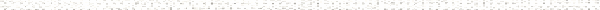A Precalculus Outline

 Chapter 5:  Further Topics in FunctionsObjective: Finding a formula for a composite functionObjective: Finding the domain of a composite functionObjective: Function notationObjective: Finding domain algebraicallyObjective: Understanding the concept of inverse functionsObjective: Identifying one-to-one functions using the Horizontal Line TestObjective: Verifying two functions are inverses using function compositionObjective: Finding a formula for an inverse functionObjective: Graphs of inverse functionsObjective: Restricting a function to make it one-to-oneObjective: Summary of Inverse FunctionsObjective: Graphing root and radical functionsObjective: Using rational exponents to simplify radical expressionsObjective: Solving equations involving radicalsObjective: Identifying extraneous solutions to equations involving radicalsObjective: Solving equations involving rational exponentsObjective: Simplifying radical expressionsObjective: Definition of Rational ExponentsObjective: Simplifying Expressions with Rational Exponents